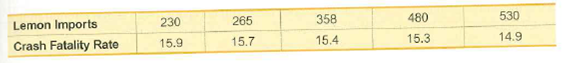# 530480358265230Lemon Imports14.915.315.415.715.9Crash Fatality Rate

Question
2 views

Lemons and Car Crashes Using the listed lemon/crash data, find the best predicted crash fatality rate for a year in which there are 500 metric tons of lemon imports. Is the prediction worthwhile?help_outlineImage Transcriptionclose530 480 358 265 230 Lemon Imports 14.9 15.3 15.4 15.7 15.9 Crash Fatality Rate fullscreen
check_circle

Step 1

Regression:

The regression analysis is conducted here by using EXCEL. The software procedure is given below:

• Enter the data.
• Select Data > Data Analysis > Regression> OK.
• Enter Input Y Range as CRASH FERTILITY RATES.
• Enter Input X Range as LEMON IMPORTS.
• Click OK.

The output using EXCEL is as follows:

Step 2

From the output, regression equation is, Crash Fatality Rate= 16.5-0.003(Lemon Imports).

Strategy for Predicting the y value:

Analyze the below mentioned conditions for predicting the y value.

• In scatterplot, the regression line fits the point well.
• The value of r indicates that there is a linear correlation between the variables.
• The prediction value is not away from the range of the given sample data.

If the regression equation satisfies the above mentioned conditions, then the regression is said to be a good model. Here, substitute the given value of x into the regression equation to predict the value of y.

If the regression equation does not satisfy the above conditions, then the regression is not a good model. Here, the best predicted value of y is the value of y-bar.

The scatter diagram, along with the best fitted line (least-squares regression line) for the data is as follows:

Step 3

The horizontal axis represents lemon imports and the vertical axis represents crash fertility rates.

From the plot, it is observed that the regression line fit the points well.

Correlation coefficient r:

The hypotheses are given below:

Null hypothesis:

H0: r = 0

That is, there is no linear correlation between the weights of lemon imports from Mexico and U.S. car fatality rates.

Alternative hypothesis:

Ha: r≠ 0

That is, there is a linear correlation between the the weights of lemon imports from Mexico and U.S. car fatality rates.

Sample correlation coefficient:

The value of the sample correlation coefficient (r) can be obtained using Excel formula.

Enter the function, =CORREL(A2:A5,B2:B5).

Thus, the value of the sample correlation coefficient, r is –0.959.

Conclusion:

The P-value is...

### Want to see the full answer?

See Solution

#### Want to see this answer and more?

Solutions are written by subject experts who are available 24/7. Questions are typically answered within 1 hour.*

See Solution
*Response times may vary by subject and question.
Tagged in

### Other